## Example Questions

1 2 3 5 Next →

### Example Question #91 : Circles

What is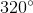in radians?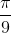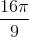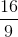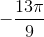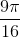Explanation:

To convert degrees to radians, we need to remember the following formula.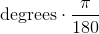.

Now lets substitute for degrees.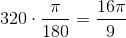### Example Question #91 : Circles

A circle is circumscribed by a square, and that square is the base of a cube. If the volume of the cube is 216, what is the area of the circle?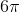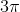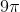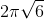Explanation:

The equation for the volume of a cube is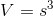If V= 216, then s = 6.

When a circle is circumscribed by a square the diameter of the circle is equal to the length of one side of the square. In this case, one side of the square is equal to 6; therefore the diameter of the circle is also 6. We can find the area of a circle with the equationand since the radius is equal to half the diameter: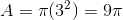1 2 3 5 Next →

### All SAT Math Resources